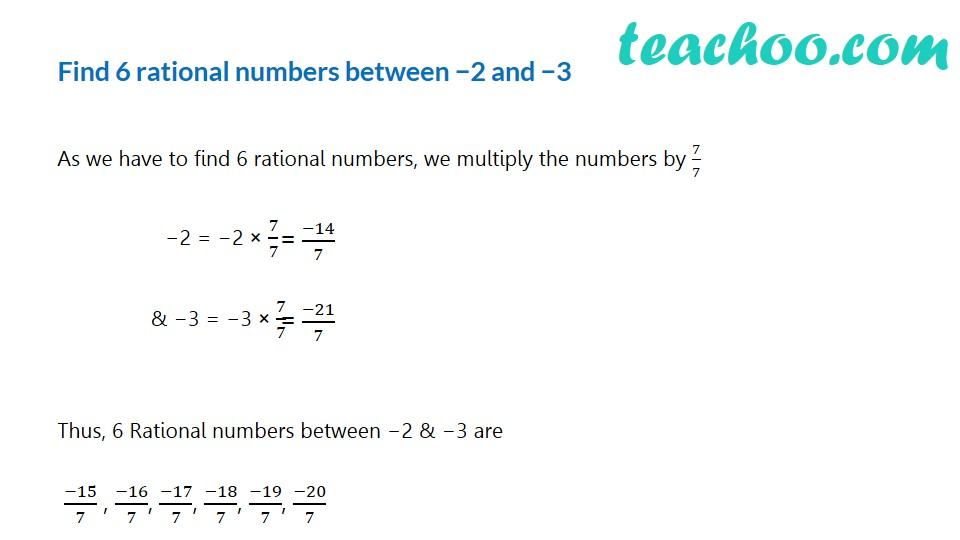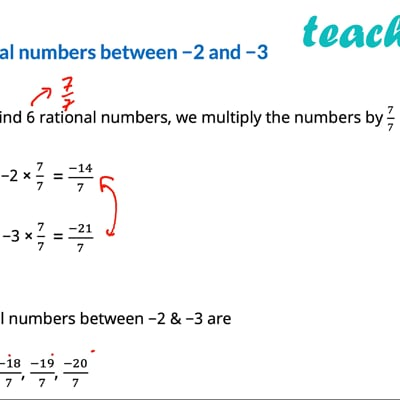Finding rational number between two numbers

Chapter 1 Class 9 Number Systems
Concept wiseThis video is only available for Teachoo black users

Introducing your new favourite teacher - Teachoo Black, at only ₹83 per month

### Transcript

Find 6 rational numbers between −2 and −3 As we have to find 6 rational numbers, we multiply the numbers by 7/7 −2 = −2 × 7/7 & −3 = −3 × 7/7 Thus, 6 Rational numbers between −2 & −3 are (−15)/7 , (−16)/7, (−17)/7, (−18)/7, (−19)/7, (−20)/7